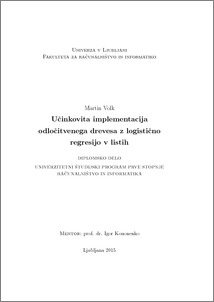# Efficient implementation of decision tree with logistic regression in leaves

Martin Volk (2015) Efficient implementation of decision tree with logistic regression in leaves. EngD thesis.Preview
PDF

## Abstract

The purpose of this thesis was implementation of an algorithm for building decision trees with logistic regression in the leaves. Since such algorithms are used for sport data analysis, where the amount of data to analyse is large, the biggest drawback of these algorithms is the consumption of computer memory required to build the model. First, an algorithm for building decision trees and an algorithm for building logistic regression models are described. This is followed by a description of an algorithm for building decision trees with logistic regression in the leaves, on which our solution is based. At the end our implementation is presented together with results of the tests.

Item Type: Thesis (EngD thesis)
Keywords: decision tree,logistic regression
Number of Pages: 48
Language of Content: Slovenian
Mentor / Comentors:
Name and SurnameIDFunction
prof. dr. Igor Kononenko237Mentor